## Tuesday, June 21, 2016

### The Backpropagation Algorithm for Artificial Neural Networks (ANNs)

In a previous post, I talked about how to use the gradient descent algorithm to optimize the weights and biases of an artificial neural network in order to give selected outputs for selected inputs. However plain gradient descent is inefficient on large neural nets since it recomputes a lot of values for each neuron, plus it has to be rewritten for every change in architecture (number of neurons and layers) and requires a lot of code. The standard solution is to use an optimized version of the gradient descent algorithm for neural nets called the backpropagation algorithm.

I will assume that you have read the previous post. Whereas the previous post was very specific using a specified architecture and a specified activation and cost function, here I will keep things as general as possible such that they can be applied on any feed forward neural network. Here are the definitions of symbols we shall be using, similar to last post's definitions:In order to help explain the algorithm and equations, I shall be applying it to the last post's architecture, just to help you understand. So here is last post's neural net: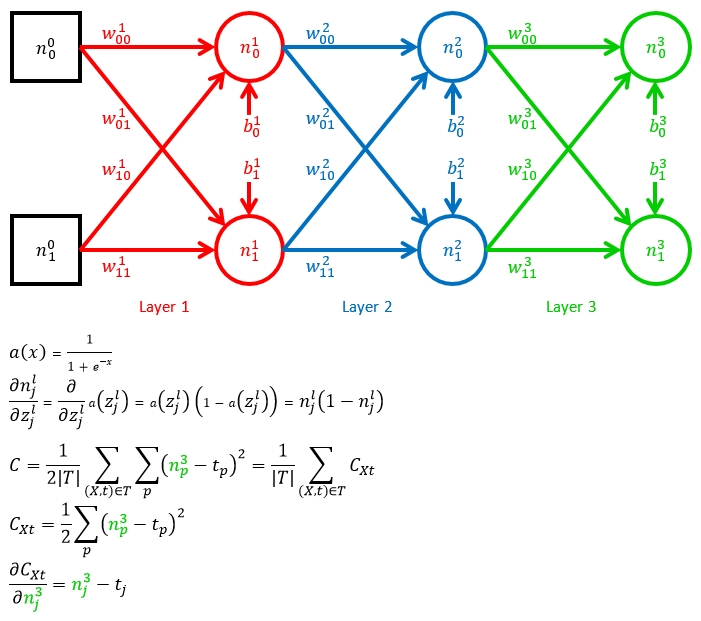In the example, we have a 3 layer neural net with 2 neurons in each layer and 2 input neurons. It uses the sigmoid function as an activation function and the mean square error as the cost function.

The main point of interest in the backpropagation algorithm is the way you find the derivative of the cost function with respect to a weight or bias in any layer. Let's look at how to find these derivatives for each layer in the example neural net.

Weights and bias in layer L (output layer)

We begin by finding the derivatives for the output layer, which are the simplest.

d Cost / d Weight L
The derivative of the cost with respect to any weight in layer L can be found using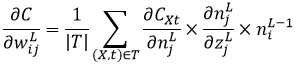Here's how we can arrive to this equation based on a weight in layer 3 in the example: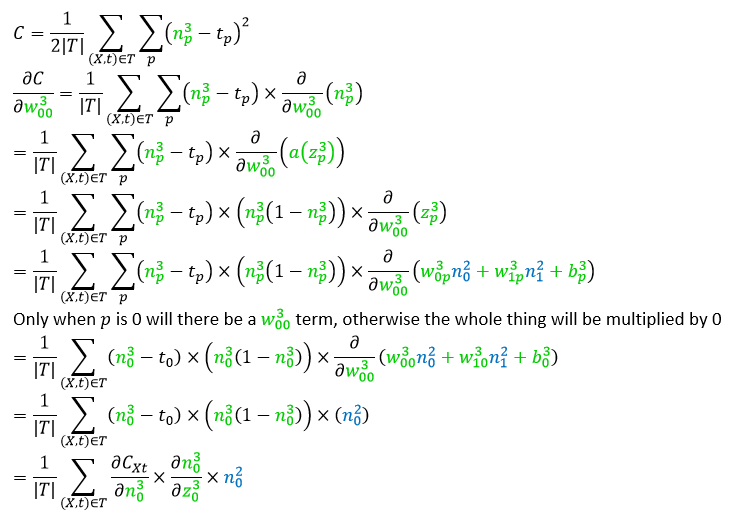d Cost / d Bias L

The derivative of the cost with respect to any bias in layer L can be found using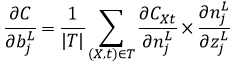Here's how we can arrive to this equation based on a bias in layer 3 in the example:Using delta

A part of the weights and biases equations is repeated. It will be convenient when finding derivatives of other layers to use an intermediate letter, called lower case delta, to represent this part of these equations.Weights and bias in layer L-1

Now we find the derivative with respect to the weights and biases in layer L-1. The derivations will be continuations of the previous derivations and we won't be repeating the first bit of the derivations again.

d Cost / d Weight L-1
The derivative of the cost with respect to any weight in layer L-1 can be found using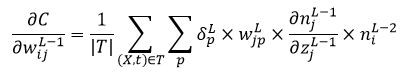Here's how we can arrive to this equation based on a weight in layer 2 in the example:d Cost / d Bias L-1

The derivative of the cost with respect to any bias in layer L-1 can be found using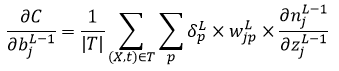Here's how we can arrive to this equation based on a bias in layer 2 in the example: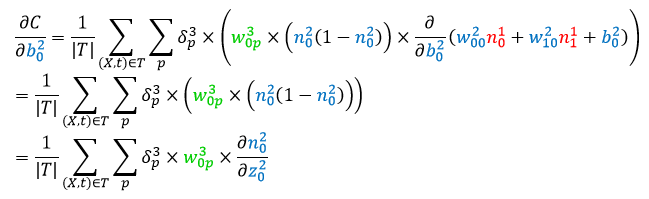Using delta

These equations can be shortened by using delta again and now we can use the previous delta to shorten this one even more.Notice how there is a recursive definition of delta. This is the basis for the backpropagation algorithm.

Weights and bias in layer L-2

Now we find the derivative with respect to the weights and biases in layer L-2. Like before, we won't be repeating the first bit of the derivations again.

d Cost / d Weight L-2
The derivative of the cost with respect to any weight in layer L-2 can be found using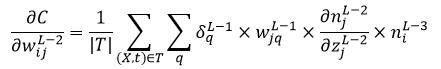Here's how we can arrive to this equation based on a weight in layer 1 in the example (notice that there is a trick we'll be using with the summations that is explained below):At the end of the derivation we did a trick with the sigmas (summations) in order to turn the equation into a recursive one.

First, we moved the delta to the inside of the sigmas. This can be done because it's just a common term that is factored out and we're factoring it back in. For example if the equation is "𝛿(a + b + c)" then we can turn that into "𝛿a + 𝛿b + 𝛿c".

Second, we swapped the "p" summation with the "q" summation. This does not change anything if you think about it. All it does is change the order of the summations, which does not change anything.

Finally we replaced the "p" summation with the previous delta, allowing us to shorten the equation.

d Cost / d Bias L-2

The derivative of the cost with respect to any bias in layer L-2 can be found usingHere's how we can arrive to this equation based on a bias in layer 1 in the example:Using delta

Again, we can shorten the equations using delta.Notice that this is exactly the same as the previous delta. This is because beyond L-1, all the deltas will be identical and can be defined using a single recursive relation.

In general: using indices

Up to now we saw what the derivatives for layer L, L-1, and L-2 are. We can now generalize these equations for any layer. Given the recursive pattern in the deltas, we can create an equation that gives the deltas of any layer provided you have the deltas of the previous layers. The base case of the recursion would be for layer L, which is defined on its own.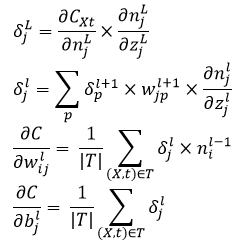Now we have a way to find the derivatives for any layer, no matter how deep the neural network is. Here is how you'd use these equations on the example: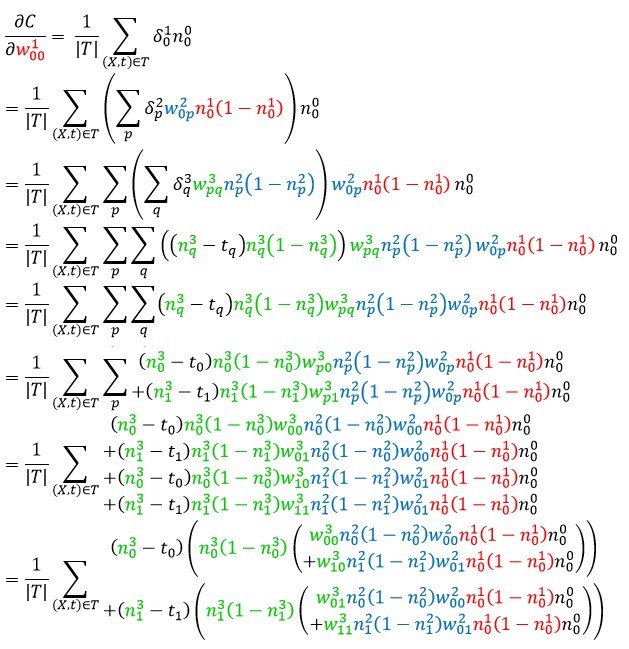In general: using matrices

As things stand, there are a lot of index letters littering our equations (i,j,p). We can get rid of them however if we use matrix operations that work on all the indices at once. If we do this we can even get shorter code when programming the backpropagation algorithm by making use of a fast matrix library such as numpy.

Using matrices in neural nets

Let's look at how we can use matrices to compute the output of a neural net. A whole layer of activations can be calculated as a whole by treating it as a vector. We'll treat vectors as being horizontal by default and which need to be transposed in order to be made vertical.

Here's an example of what we want to calculate: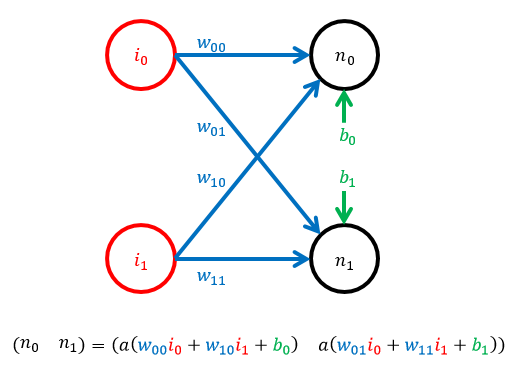Of course we're still using indices just because we're organising our activations in a vector. In order to get rid of the indices we need to treat the weights as a matrix, where the first index is the row number and the second index is the column number. That way we can have a single letter "w" which represents all of the weights of a layer. When multiplied by a vector of the previous layer's activations, the matrix multiplication will result in another vector of weighted sums which can be added to a bias vector and passed through an activation function to compute the next vector of activations. Here is an example of the calculation based on the above example:Final clean generalized deltas and cost gradients

And now we can finally see what the clean matrix-based general derivatives are for any layer: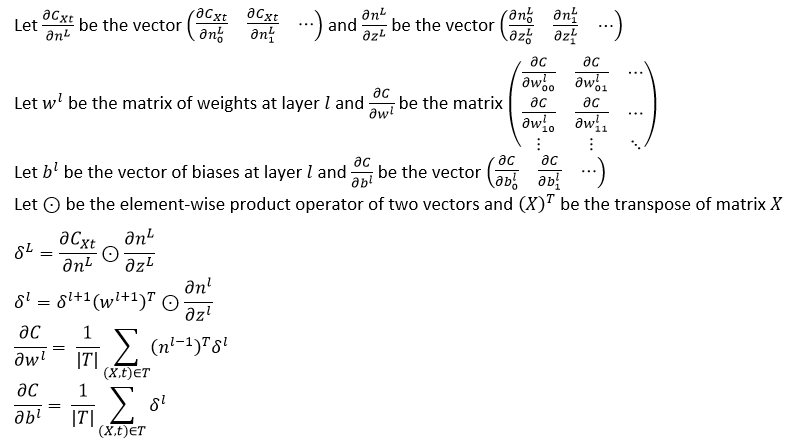The backpropagation algorithm

Finding the gradients is one step (the most complex one) of the backpropagation algorithm. The full backpropagation algorithm goes as follows:

1. For each input-target pair in the training set,
1. Compute the activations and the "z" of each layer when passing the input through the network. This is called a forward pass.
2. Use the values collected from the forward pass to calculate the gradient of the cost with respect to each weight and bias, starting from the output layer and using the delta equations to compute the gradients for previous layers. This is called the backward pass.
2. Following the backward pass, you have the gradient of the cost with respect to each weight and bias for each input in the training set. Add up all the corresponding gradients of each input in the training set (all the gradients with respect to the same weight or bias).
3. Now use the gradient to perform gradient descent by multiplying the gradient by a step size, or learning rate as it's called in the backpropagation algorithm, and subtracting it from the corresponding weights and biases. In algebra,
weight = weight - learningrate*dCost/dWeight

In code
And this is what the full program looks like in Python 3 using numpy to perform matrix operations. Again, we shall be training the neural net to perform the function of a half adder. A half adder adds together two binary digits and returns the sum and carry. So 0 + 1 in binary gives 1 carry 0 whilst 1 + 1 in binary gives 0 carry 1.

import math, random
import numpy as np

class ANN:
'''General artificial neural network class.'''

def __init__(self, num_in, num_hiddens, num_out, trainingset):
'''Create a neural network with a set number of layers and neurons and with initialised weights and biases.'''
if not all(len(x)==num_in and len(y)==num_out for (x,y) in trainingset.items()):
raise ValueError()

self._num_in = num_in
self._num_hiddens = num_hiddens
self._num_out = num_out
self._trainingset = trainingset

self._num_layers = len(num_hiddens) + 1

self._weights = dict([
(1, np.array([
[self.weight_init(1, i, j) for j in range(num_hiddens)]
for i in range(num_in)
]))
]
+ [
(l+1, np.array([
[self.weight_init(l+1, i, j) for j in range(num_hiddens[l])]
for i in range(num_hiddens[l-1])
]))
for l in range(1, len(num_hiddens))
]
+ [
(self._num_layers, np.array([
[self.weight_init(self._num_layers, i, j) for j in range(num_out)]
for i in range(num_hiddens[-1])
]))
])

self._biases = dict([
(l+1, np.array([
self.bias_init(l+1, j) for j in range(num_hiddens[l])
]))
for l in range(len(num_hiddens))
]
+ [
(self._num_layers, np.array([
self.bias_init(self._num_layers, j) for j in range(num_out)
]))
])

def weight_init(self, layer, i, j):
'''How to initialise weights.'''
return 2*random.random()-1

def bias_init(self, layer, j):
'''How to initialise biases.'''
return 2*random.random()-1

def a(self, z):
'''The activation function.'''
return 1/(1 + np.vectorize(np.exp)(-z))

def da_dz(self, z):
'''The derivative of the activation function.'''
return self.a(z)*(1-self.a(z))

def out(self, x):
'''Compute the output of the neural network given an input vector.'''
n = x
for l in range(1, self._num_layers+1):
n = self.a(np.dot(n, self._weights[l]) + self._biases[l])
return n

def cost(self):
'''The cost function based on the training set.'''
return sum(sum((self.out(x) - t)**2) for (x,t) in self._trainingset.items())/(2*len(self._trainingset))

def dCxt_dnL(self, x, t):
'''The derivative of the cost function for a particular input-target pair with respect to the output of the network.'''
return self.out(x) - t

'''Calculate and return the gradient of the cost function with respect to each weight and bias in the network.'''
dC_dws = dict()
dC_dbs = dict()
for l in range(1, self._num_layers+1):
dC_dws[l] = np.zeros_like(self._weights[l])
dC_dbs[l] = np.zeros_like(self._biases[l])

for (x,t) in self._trainingset.items():
#forward pass
zs = dict()
ns = dict()
ns_T = dict()
ns = np.array(x)
ns_T = np.array([np.array(x)]).T
for l in range(1, self._num_layers+1):
z = np.dot(ns[l-1], self._weights[l]) + self._biases[l]
zs[l] = z
n = self.a(z)
ns[l] = n
ns_T[l] = np.array([n]).T

#backward pass
d = self.dCxt_dnL(x, t)*self.da_dz(zs[self._num_layers])
dC_dws[self._num_layers] += np.dot(ns_T[self._num_layers-1], np.array([d]))
dC_dbs[self._num_layers] += d
for l in range(self._num_layers-1, 1-1, -1):
d = np.dot(d, self._weights[l+1].T)*self.da_dz(zs[l])
dC_dws[l] += np.dot(ns_T[l-1], np.array([d]))
dC_dbs[l] += d

for l in range(1, self._num_layers+1):
dC_dws[l] /= len(self._trainingset)
dC_dbs[l] /= len(self._trainingset)

return (dC_dws, dC_dbs)

def epoch_train(self, learning_rate):
'''Train the neural network for one epoch.'''
for l in range(1, self._num_layers+1):
self._weights[l] -= learning_rate*dC_dws[l]
self._biases[l] -= learning_rate*dC_dbs[l]

'''Check if the gradients are being calculated correctly. This is done according to http://ufldl.stanford.edu/tutorial/supervised/DebuggingGradientChecking/ . This method calculates the difference between each calculated gradient (according to get_cost_gradients()) and an estimated gradient using a small number epsilon. If the gradients are calculated correctly then the returned numbers should all be very small (smaller than 1e-10.'''

approx_dC_dws = dict()
approx_dC_dbs = dict()
for l in range(1, self._num_layers+1):
approx_dC_dws[l] = np.zeros_like(self._weights[l])
approx_dC_dbs[l] = np.zeros_like(self._biases[l])

for l in range(1, self._num_layers+1):
(rows, cols) = self._weights[l].shape
for r in range(rows):
for c in range(cols):
orig = self._weights[l][r][c]
self._weights[l][r][c] = orig + epsilon
cost_plus = self.cost()
self._weights[l][r][c] = orig - epsilon
cost_minus = self.cost()
self._weights[l][r][c] = orig
approx_dC_dws[l][r][c] = (cost_plus - cost_minus)/(2*epsilon)

(cols,) = self._biases[l].shape
for c in range(cols):
orig = self._biases[l][c]
self._biases[l][c] = orig + epsilon
cost_plus = self.cost()
self._biases[l][c] = orig - epsilon
cost_minus = self.cost()
self._biases[l][c] = orig
approx_dC_dbs[l][c] = (cost_plus - cost_minus)/(2*epsilon)

errors_w = dict()
errors_b = dict()
for l in range(1, self._num_layers+1):
errors_w[l] = abs(predicted_dC_dws[l] - approx_dC_dws[l])
errors_b[l] = abs(predicted_dC_dbs[l] - approx_dC_dbs[l])
return (errors_w, errors_b)

################################################################
nn = ANN(
2, #number of inputs
[ 2, 2 ], #number of hidden neurons (each number is a hidden layer)
2, #number of outputs
{ #training set
(0,0):(0,0),
(0,1):(1,0),
(1,0):(1,0),
(1,1):(0,1)
}
)

print('Initial cost:', nn.cost())
print('Starting training')
for epoch in range(1, 20000+1):
nn.epoch_train(0.5)
if epoch%1000 == 0:
print(' Epoch:', epoch, ', Current cost:', nn.cost())
print('Training finished')

print('Neural net output:')
for (x,t) in sorted(nn._trainingset.items()):
print(' ', x, ':', nn.out(x))


After 20,000 iterations I got this output:
Initial cost: 0.232581149941
Starting training
Epoch: 1000 , Current cost: 0.218450291043
Epoch: 2000 , Current cost: 0.215777328824
Epoch: 3000 , Current cost: 0.178593050179
Epoch: 4000 , Current cost: 0.102704613785
Epoch: 5000 , Current cost: 0.0268721186425
Epoch: 6000 , Current cost: 0.00819827730488
Epoch: 7000 , Current cost: 0.00444660869981
Epoch: 8000 , Current cost: 0.00298786209459
Epoch: 9000 , Current cost: 0.00223137023455
Epoch: 10000 , Current cost: 0.00177306322414
Epoch: 11000 , Current cost: 0.00146724847349
Epoch: 12000 , Current cost: 0.00124934223594
Epoch: 13000 , Current cost: 0.00108652952015
Epoch: 14000 , Current cost: 0.000960438140866
Epoch: 15000 , Current cost: 0.000860007442299
Epoch: 16000 , Current cost: 0.000778192177283
Epoch: 17000 , Current cost: 0.000710297773182
Epoch: 18000 , Current cost: 0.000653078479503
Epoch: 19000 , Current cost: 0.000604220195232
Epoch: 20000 , Current cost: 0.0005620295804
Training finished
Neural net output:
(0, 0) : [ 0.02792593  0.00058427]
(0, 1) : [ 0.97284142  0.01665468]
(1, 0) : [ 0.97352071  0.01661805]
(1, 1) : [ 0.03061993  0.97196112]